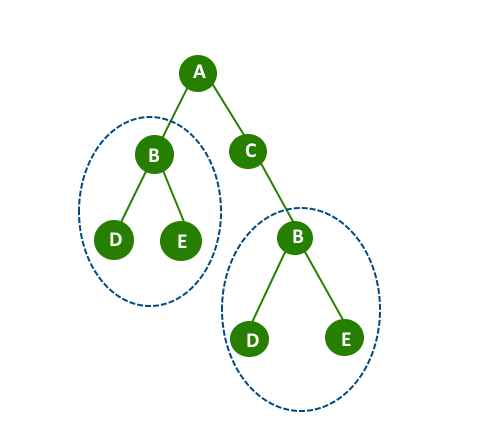# [LeetCode From Day One] - 572. Subtree of Another tree

3             4
/  \           /   \
4       5     1     2
/   \
1     2
Output: true

s的子树由s中的任意一个节点以及该节点的所有子孙节点组成。s本身也是s的子树。## 遍历s

class Solution {
public boolean isSubtree(TreeNode s, TreeNode t) {
if(s == null) return false;
return isEqual(s, t) || isSubtree(s.left, t) || isSubtree(s.right, t);
}

private boolean isEqual(TreeNode s, TreeNode t) {
if(s == null && t == null) return true;
if(s != null && t == null || s== null && t != null) return false;
if(s.val == t.val) return isEqual(s.left, t.left) && isEqual(s.right, t.right);
return false;
}
}


## 前序遍历s与t

     1         1
/           \
1             1


class Solution {
public boolean isSubtree(TreeNode s, TreeNode t) {
String pres = preorder(s, true);
String pret = preorder(t, true);

return pres.indexOf(pret) >= 0;
}

private String preorder(TreeNode n, boolean isLeft) {
if(n == null) {
if(isLeft) return "lnull";
else return "rnull";
}
return "#" + n.val + preorder(n.left, true) + preorder(n.right, false);
}
}